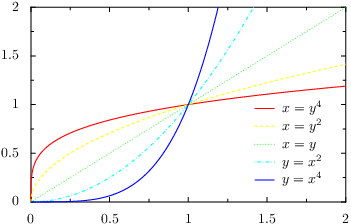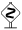# PyX — Example: graphs/change.py

0.6 KB
69.2 KB
59.8 KB
28.4 KB
45.3 KB

## Plotting more than one data set and adding a graph key```from pyx import *

g = graph.graphxy(width=8,
x=graph.axis.linear(min=0, max=2),
y=graph.axis.linear(min=0, max=2),
key=graph.key.key(pos="br", dist=0.1))
g.plot([graph.data.function("x(y)=y**4", title=r"\$x = y^4\$"),
graph.data.function("x(y)=y**2", title=r"\$x = y^2\$"),
graph.data.function("x(y)=y", title=r"\$x = y\$"),
graph.data.function("y(x)=x**2", title=r"\$y = x^2\$"),
graph.data.function("y(x)=x**4", title=r"\$y = x^4\$")],
g.writeEPSfile("change")
g.writePDFfile("change")
g.writeSVGfile("change")
```

### Description

In this example we demonstrate how to plot more than one data set in a graph. Furthermore, adding a graph key labelling the data is explained.

In order to display more than one data set, we pass a list of data sets to the `plot` method of the graph. In the present case, we contruct this list out of three lists of `graph.data.function` instances. For each data set we also set a title using the corresponding keyword argument of the data class.

For a more colorful output, we pass the `color.gradient.Rainbow` color gradient to the `graph.style.line` class. PyX then automatically chooses color spanning the whole range of the rainbow gradient.If you look at the output, you will notice that not only the colors are cycled through, but also the line style changes. The reason for this behavior is that the default line attributes of the `graph.style.line` style contain an `attr.changelist` comprising a couple of different line styles. When you pass additional line attributes, these are appended to the default attributes (this is generally the case in PyX). Consequently, you get a change of both the color and the line style. If you only want to change the color, either pass the desired line style explicitly, i.e., use

```graph.style.line([style.linestyle.solid, color.gradient.Rainbow])
```

or clear a previous setting of the line style using the `clear` attribute of the corresponding class:

```graph.style.line([style.linestyle.clear, color.gradient.Rainbow])
```It is also possible to call the `plot` method several times. Note that the changing of the styles is also performed when the style instances are used on several plot commands within the same graph as long as the same set of style instances are passed at the `plot` method calls.

Finally, when plotting more than one data set you might want to label them in your graph. We already have passed titles identifying each data set, so we only have to add a graph key. This is done by passing a `graph.key.key` instance as the `key` keyword argument to the graph. Usually, you only need to specify the position of the key using the `pos` argument of the `graph.key.key` constructor. The syntax should be self-explaining; here we use `br` to put the key at the bottom-right position of the graph. In order to save some space, we furthermore decreases the distance between the key entries a little bit by passing a value of 0.1 to the `dist` argument.